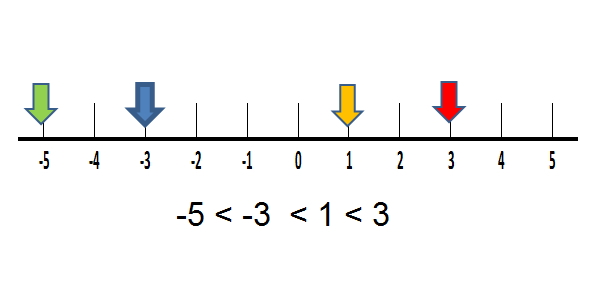# Comparing And Ordering Integers Quiz: Test!

9 Questions | Attempts: 1754Settings.

• 1.
Integers are whole numbers that describe opposite ideas in mathematics.
• A.

True

• B.

False

• 2.
Absolute value measures the:
• A.

Opposite

• B.

Distance of a number to 0

• C.

GCF

• D.

LCM

• 3.
What is used to represent about sitting at the sea level?
• A.

Positive Number

• B.

Negative Number

• C.

0

• 4.
What integer represents a loss of 10 pounds?
• A.

10

• B.

-10

• C.

0

• 5.
Which would NOT be represented by a negative number?
• A.

Below sea level

• B.

A decrease in temperature

• C.

A withdrawal of money

• D.

An increase in pay rate

• 6.
Monday's temperature was -3°C and Tuesdays was -5°C. Which day was colder?
• A.

Neither they were equal

• B.

Monday

• C.

Tuesday

• D.

Friday

• 7.
Which of the following are arranged from least to greatest?
• A.

-4, -6, 2, 4

• B.

2, 0, 1, 3, 5

• C.

-8, -1, 3, 7, 5

• D.

-8, -5, -3, -1

• 8.
Which symbol will make the sentence true? | 4 - 3 | ___ 1
• A.

>

• B.

<

• C.

=

• 9.
Arrange the given set of integers in ascending order. { -1, 3, -5, -7 }
• A.

{ -1, 3, -5, -7 }

• B.

{ -7, -5, -1, 3 }

• C.

{ -1, -5, -7, 3 }

• D.

{ -5, -7, -1, 3 }

## Related TopicsBack to top
×

Wait!
Here's an interesting quiz for you.